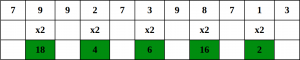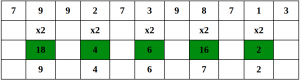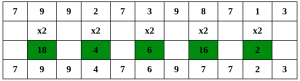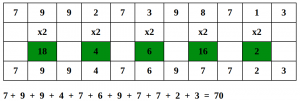GeeksforGeeks App
Open AppBrowser
Continue

# Luhn algorithm

The Luhn algorithm, also known as the modulus 10 or mod 10 algorithm, is a simple checksum formula used to validate a variety of identification numbers, such as credit card numbers, IMEI numbers, Canadian Social Insurance Numbers. The LUHN formula was created in the late 1960s by a group of mathematicians. Shortly thereafter, credit card companies adopted it. Because the algorithm is in the public domain, it can be used by anyone. Most credit cards and many government identification numbers use the algorithm as a simple method of distinguishing valid numbers from mistyped or otherwise incorrect numbers. It was designed to protect against accidental errors, not malicious attacks.

#### Steps involved in the Luhn algorithm

Let’s understand the algorithm with an example:
Consider the example of an account number “79927398713“.

Step 1 – Starting from the rightmost digit, double the value of every second digit,Step 2 – If doubling of a number results in a two digit number i.e greater than 9(e.g., 6 × 2 = 12), then add the digits of the product (e.g., 12: 1 + 2 = 3, 15: 1 + 5 = 6), to get a single digit number.Step 3 – Now take the sum of all the digits.Step 4 – If the total modulo 10 is equal to 0 (if the total ends in zero) then the number is valid according to the Luhn formula; else it is not valid.Since the sum is 70 which is a multiple of 10, the account number is possibly valid.

The idea is simple; we traverse from the end. For every second digit, we double it before adding it. We add two digits of the number obtained after doubling.

Implementation:

## C++

 `// C++ program to implement Luhn algorithm``#include ``using` `namespace` `std;` `// Returns true if given card number is valid``bool` `checkLuhn(``const` `string& cardNo)``{``    ``int` `nDigits = cardNo.length();` `    ``int` `nSum = 0, isSecond = ``false``;``    ``for` `(``int` `i = nDigits - 1; i >= 0; i--) {` `        ``int` `d = cardNo[i] - ``'0'``;` `        ``if` `(isSecond == ``true``)``            ``d = d * 2;` `        ``// We add two digits to handle``        ``// cases that make two digits after``        ``// doubling``        ``nSum += d / 10;``        ``nSum += d % 10;` `        ``isSecond = !isSecond;``    ``}``    ``return` `(nSum % 10 == 0);``}` `// Driver code``int` `main()``{``    ``string cardNo = ``"79927398713"``;``    ``if` `(checkLuhn(cardNo))``        ``printf``(``"This is a valid card"``);``    ``else``        ``printf``(``"This is not a valid card"``);``    ``return` `0;``}`

## Java

 `// Java program to implement``// Luhn algorithm``import` `java.io.*;` `class` `GFG {``    ` `// Returns true if given``// card number is valid``static` `boolean` `checkLuhn(String cardNo)``{``    ``int` `nDigits = cardNo.length();` `    ``int` `nSum = ``0``;``    ``boolean` `isSecond = ``false``;``    ``for` `(``int` `i = nDigits - ``1``; i >= ``0``; i--)``    ``{` `        ``int` `d = cardNo.charAt(i) - ``'0'``;` `        ``if` `(isSecond == ``true``)``            ``d = d * ``2``;` `        ``// We add two digits to handle``        ``// cases that make two digits``        ``// after doubling``        ``nSum += d / ``10``;``        ``nSum += d % ``10``;` `        ``isSecond = !isSecond;``    ``}``    ``return` `(nSum % ``10` `== ``0``);``}` `    ``// Driver code``    ``static` `public` `void` `main (String[] args)``    ``{``        ``String cardNo = ``"79927398713"``;``        ``if` `(checkLuhn(cardNo))``            ``System.out.println(``"This is a valid card"``);``        ``else``            ``System.out.println(``"This is not a valid card"``);``    ` `    ``}``}` `// This Code is contributed by vt_m.`

## Python3

 `# Python3 program to implement``# Luhn algorithm` `# Returns true if given card``# number is valid``def` `checkLuhn(cardNo):``    ` `    ``nDigits ``=` `len``(cardNo)``    ``nSum ``=` `0``    ``isSecond ``=` `False``    ` `    ``for` `i ``in` `range``(nDigits ``-` `1``, ``-``1``, ``-``1``):``        ``d ``=` `ord``(cardNo[i]) ``-` `ord``(``'0'``)``    ` `        ``if` `(isSecond ``=``=` `True``):``            ``d ``=` `d ``*` `2`` ` `        ``# We add two digits to handle``        ``# cases that make two digits after``        ``# doubling``        ``nSum ``+``=` `d ``/``/` `10``        ``nSum ``+``=` `d ``%` `10`` ` `        ``isSecond ``=` `not` `isSecond``    ` `    ``if` `(nSum ``%` `10` `=``=` `0``):``        ``return` `True``    ``else``:``        ``return` `False` `# Driver code  ``if` `__name__``=``=``"__main__"``:``    ` `    ``cardNo ``=` `"79927398713"``    ` `    ``if` `(checkLuhn(cardNo)):``        ``print``(``"This is a valid card"``)``    ``else``:``        ``print``(``"This is not a valid card"``)` `# This code is contributed by rutvik_56`

## C#

 `// C# program to implement``// Luhn algorithm``using` `System;` `class` `GFG {``    ` `// Returns true if given``// card number is valid``static` `bool` `checkLuhn(String cardNo)``{``    ``int` `nDigits = cardNo.Length;` `    ``int` `nSum = 0;``    ``bool` `isSecond = ``false``;``    ``for` `(``int` `i = nDigits - 1; i >= 0; i--)``    ``{` `         ``int` `d = cardNo[i] - ``'0'``;` `        ``if` `(isSecond == ``true``)``            ``d = d * 2;` `        ``// We add two digits to handle``        ``// cases that make two digits``        ``// after doubling``        ``nSum += d / 10;``        ``nSum += d % 10;` `        ``isSecond = !isSecond;``    ``}``    ``return` `(nSum % 10 == 0);``}` `    ``// Driver code``    ``static` `public` `void` `Main()``    ``{``        ``String cardNo = ``"79927398713"``;``        ``if` `(checkLuhn(cardNo))``            ``Console.WriteLine(``"This is a valid card"``);``        ``else``            ``Console.WriteLine(``"This is not a valid card"``);``    ` `    ``}``}` `// This Code is contributed by vt_m.`

## Javascript

 ``

Output

`This is a valid card`

The Luhn algorithm detects any single-digit error, as well as almost all transpositions of adjacent digits.

This article is contributed by Vishal Kumar Gupta. If you like GeeksforGeeks and would like to contribute, you can also write an article using write.geeksforgeeks.org or mail your article to review-team@geeksforgeeks.org. See your article appearing on the GeeksforGeeks main page and help other Geeks.

My Personal Notes arrow_drop_up